# Math in Focus Grade 5 Chapter 3 Practice 6 Answer Key Subtracting Mixed Numbers

Go through the Math in Focus Grade 5 Workbook Answer Key Chapter 3 Practice 6 Subtracting Mixed Numbers to finish your assignments.

## Math in Focus Grade 5 Chapter 3 Practice 6 Answer Key Subtracting Mixed Numbers

Subtract. Express each difference in simplest form.

Example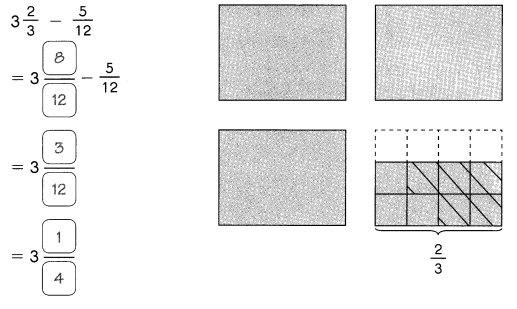Question 1.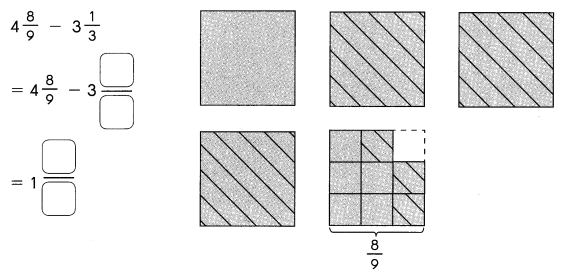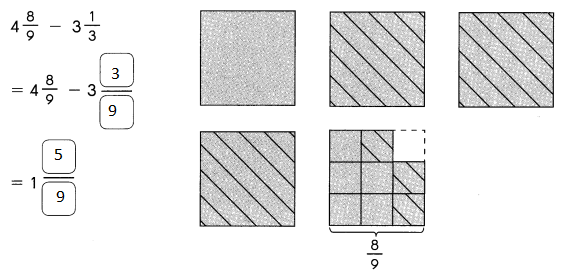Explanation:
The difference is found between two mixed fractions
as the denominators are same we directly subtracted the fraction

Subtract. Express each difference ¡n simplest form.

Question 2.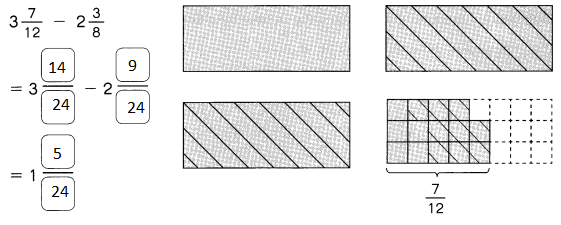Explanation:
The difference is found between two mixed fractions
as the denominators are different we changed them to like denominators
then subtracted.

Question 3.
3$$\frac{5}{9}$$ – 1$$\frac{1}{2}$$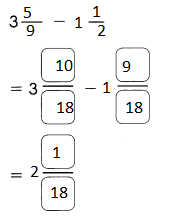Explanation:
The difference is found between two mixed fractions
as the denominators are different we changed them to like denominators
then subtracted.

Question 4.
7$$\frac{5}{6}$$ – 2$$\frac{1}{4}$$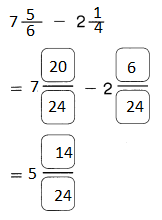Explanation:
The difference is found between two mixed fractions
as the denominators are different we changed them to like denominators
then subtracted.

Subtract. Express each difference as a mixed number.

Question 5.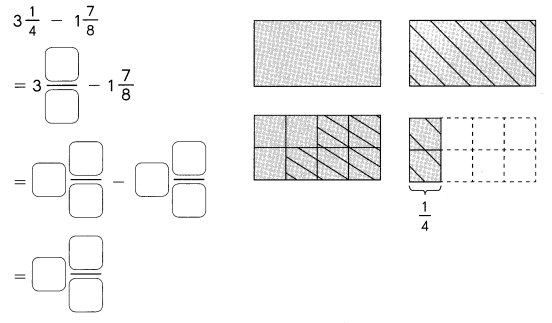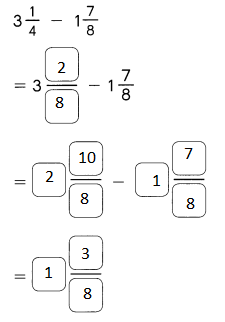Explanation:
The difference is found between two mixed fractions
as the denominators are different we changed them to like denominators
then we are unable to subtract the mixed fractions
so we converted the numerator as that difference can be done

Subtract. Express each difference as a mixed number.

Question 6.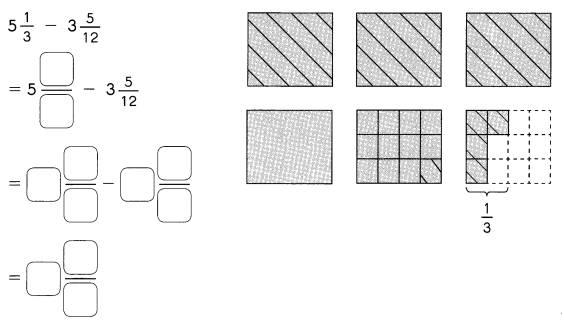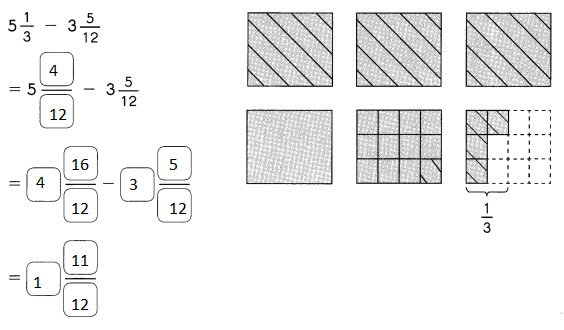Explanation:
The difference is found between two mixed fractions
as the denominators are different we changed them to like denominators
then we are unable to subtract the mixed fractions
so we converted the numerator as that difference can be done

Question 7.
4$$\frac{1}{5}$$ – 1$$\frac{1}{3}$$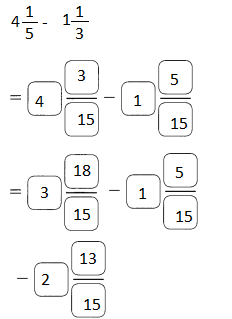Explanation:
The difference is found between two mixed fractions
as the denominators are different we changed them to like denominators
then we are unable to subtract the mixed fractions
so we converted the numerator as that difference can be done

Question 8.
6$$\frac{3}{8}$$ – 3$$\frac{5}{6}$$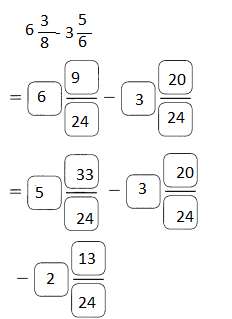Explanation:
The difference is found between two mixed fractions
as the denominators are different we changed them to like denominators
then we are unable to subtract the mixed fractions
so we converted the numerator as that difference can be done

Question 9.
7$$\frac{1}{4}$$ – 5$$\frac{11}{12}$$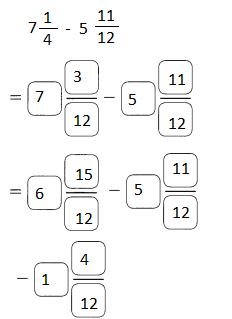Explanation:
The difference is found between two mixed fractions
as the denominators are different we changed them to like denominators
then we are unable to subtract the mixed fractions
so we converted the numerator as that difference can be done

Question 10.
8$$\frac{1}{3}$$ – 4$$\frac{3}{4}$$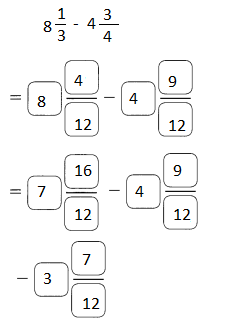Explanation:
The difference is found between two mixed fractions
as the denominators are different we changed them to like denominators
then we are unable to subtract the mixed fractions
so we converted the numerator as that difference can be done

Use benchmarks to estimate each difference.

Example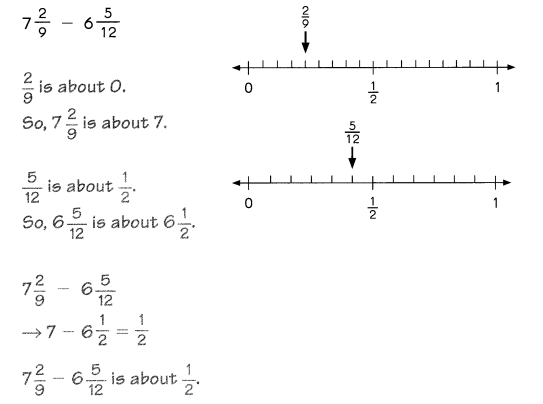Question 11.
12$$\frac{2}{5}$$ – 8$$\frac{7}{12}$$
12$$\frac{2}{5}$$ – 8$$\frac{7}{12}$$ = 4
Explanation:
$$\frac{2}{5}$$ is about $$\frac{1}{2}$$
so, 12$$\frac{2}{5}$$  is about 12$$\frac{1}{2}$$
$$\frac{7}{12}$$ is about $$\frac{1}{2}$$
so, 8$$\frac{7}{12}$$  is about 8$$\frac{1}{2}$$
12$$\frac{1}{2}$$ – 8$$\frac{1}{2}$$ = 4

Question 12.
20$$\frac{1}{8}$$ – 5$$\frac{3}{9}$$
20$$\frac{1}{8}$$ – 5$$\frac{3}{9}$$ = 15
$$\frac{1}{8}$$ is about 0
$$\frac{3}{9}$$ is about 0